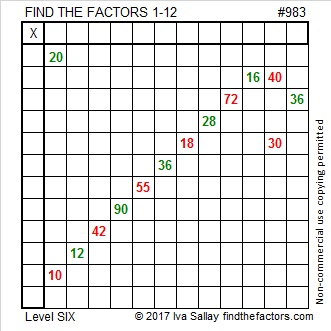# 1571 Candy Cane

### Today’s Puzzle:

The shepherds’ crooks from that first Christmas night have become the sweet candy canes we often see on today’s Christmas trees. Can you find the factors from 1 to 12 that will make this mystery level puzzle function like a multiplication table? Remember to use logic to find the factors.### Factors of 1571:

• 1571 is a prime number.
• Prime factorization: 1571 is prime.
• 1571 has no exponents greater than 1 in its prime factorization, so √1571 cannot be simplified.
• The exponent in the prime factorization is 1. Adding one to that exponent we get (1 + 1) = 2. Therefore 1571 has exactly 2 factors.
• The factors of 1571 are outlined with their factor pair partners in the graphic below.How do we know that 1571 is a prime number? If 1571 were not a prime number, then it would be divisible by at least one prime number less than or equal to √1571. Since 1571 cannot be divided evenly by 2, 3, 5, 7, 11, 13, 17, 19, 23, 29, 31, or 37, we know that 1571 is a prime number.

### More about the Number 1571:

1571 is the sum of two consecutive numbers:
785 + 786 = 1571.

1571 is also the difference of two squares:
786² – 785² = 1571.

Do you see the relationship between those two facts?

# 1318 Another Candy Cane

Candy canes are rarely alone. They almost always have a twin close-by. Nevertheless, this mystery-level candy cane puzzle only looks similar to the previous one. You will have to consider completely different factors to solve it.

Print the puzzles or type the solution in this excel file: 12 factors 1311-1319

Here are a few facts about the number 1318:

• 1318 is a composite number.
• Prime factorization: 1318 = 2 × 659
• The exponents in the prime factorization are 1 and 1. Adding one to each and multiplying we get (1 + 1)(1 + 1) = 2 × 2 = 4. Therefore 1318 has exactly 4 factors.
• Factors of 1318: 1, 2, 659, 1318
• Factor pairs: 1318 = 1 × 1318 or 2 × 659
• 1318 has no square factors that allow its square root to be simplified. √1318 ≈ 36.30427

1318 is palindrome 414 in BASE 18

# 1317 Candy Cane

Candy canes are a sweet tradition every Christmas. This mystery level puzzle won’t be easy, but it can be a sweet way to remember all the multiplication and division facts needed to solve it.

Print the puzzles or type the solution in this excel file: 12 factors 1311-1319

Now I’ll write a few facts about the number 1317:

• 1317 is a composite number.
• Prime factorization: 1317 = 3 × 439
• The exponents in the prime factorization are 1 and 1. Adding one to each and multiplying we get (1 + 1)(1 + 1) = 2 × 2 = 4. Therefore 1317 has exactly 4 factors.
• Factors of 1317: 1, 3, 439, 1317
• Factor pairs: 1317 = 1 × 1317 or 3 × 439
• 1317 has no square factors that allow its square root to be simplified. √1317 ≈ 36.29049

1317 is a palindrome in several bases:
It’s 10100100101 in BASE 2,
919 in BASE 12,
525 in BASE 16, and
1J1 in BASE 28,

# 983 Candy Cane

Candy canes have been a part of the Christmas season for ages. Here’s a candy cane puzzle for you to try. It’s a level 6 so it won’t be easy, but you will taste its sweetness once you complete it. Go ahead and get started!Print the puzzles or type the solution in this excel file: 12 factors 978-985

Here’s some information about prime number 983:

983 is the sum of consecutive prime numbers two different ways:
It is the sum of the seventeen prime numbers from 23 to 97.
It is also the sum of the thirteen prime numbers from 47 to 103.

• 983 is a prime number.
• Prime factorization: 983 is prime.
• The exponent of prime number 983 is 1. Adding 1 to that exponent we get (1 + 1) = 2. Therefore 983 has exactly 2 factors.
• Factors of 983: 1, 983
• Factor pairs: 983 = 1 × 983
• 983 has no square factors that allow its square root to be simplified. √983 ≈ 31.35283

How do we know that 983 is a prime number? If 983 were not a prime number, then it would be divisible by at least one prime number less than or equal to √983 ≈ 31.4. Since 983 cannot be divided evenly by 2, 3, 5, 7, 11, 13, 17, 19, 23, 29 or 31, we know that 983 is a prime number.